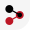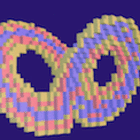# DyToComp 2018

The conference is devoted to computational aspects of dynamics and topology. The themes of the conference include, but are not limited to:

• Dynamics and Computation
• classical numerical methods for dynamical ODEs and PDEs,
• algorithms for rigorous numerics of dynamical systems,
• KAM theory and rigorous numerics,
• variational methods,
• applications to celestial mechanics,
• numerical experiments in dynamics.
• Topology and Computation
• homology algorithms,
• persistent homology,
• data analysis by topological methods,
• topological methods in computer science,
• topological methods in shape analysis, imaging and computer vision,
• statistical topology,
• directed algebraic topology and concurrency,
• combinatorial methods in applied algebraic topology,
• topological aspects of computational algebra.
• Dynamics and Topology
• topological invariants of dynamical systems, Conley index,
• combinatorial topological dynamics,
• topological criteria for qualitative analysis of dynamical systems,
• topological methods of dynamical systems as tools in developing numerical algorithms,
• numerical algorithms for topological invariants of dynamical systems.